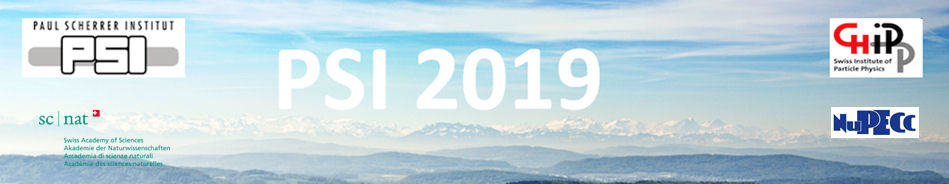#Physics of fundamental Symmetries and Interactions - PSI2019

Oct 20 – 25, 2019
PSI
Europe/Zurich timezone

## Precision measurements in molecular hydrogen

Oct 25, 2019, 11:10 AM
30m
WHGA/001 - Auditorium (PSI)

Invited Talk

### Speaker

Frédéric Merkt (ETH Zurich, Laboratory of Physical Chemistry)

### Description

Few-electron molecules are attractive systems for precision spectroscopy because their properties can be calculated with high accuracy by quantum-chemical methods.$^{1,2,3}$ The measurements serve to test theoretical predictions, ideally at the level where their accuracy is limited by the uncertainties of the fundamental constants or by unrecognized physical effects. I will report on precision measurements of energy intervals in cold samples of H$_2$. In particular, we determine the ionization energy with a precision ($\Delta\nu/\nu$) of 10$^{-10}$ from high-resolution Rydberg spectra$^{4,5,6}$ and derive the dissociation energy with an accuracy of 350 kHz, approaching the level where the size of the proton and the uncertainty in the proton-to-electron mass ratio would limit the accuracy of otherwise exact calculations. Comparison will be made to recent theoretical results in the context of a more-than-100-year-long series of experimental and theoretical determinations of the dissociation energy of H$_2$. I will also discuss the determination of an upper bound for a hypothetical global shift of the energy level structure of ortho-H$_2$ with respect to that of para-H$_2$.

1. V. I. Korobov, L. Hilico and J.-P. Karr, Phys. Rev. Lett. 118, 233001 (2017).
2. M. Puchalski, J. Komasa, P. Czachorowski, and K. Pachucki, Phys. Rev. Lett. 122, 103003 (2019).
3. E. Mátyus, J. Chem. Phys. 149, 194112 (2018).
4. M. Beyer, N. Hölsch, J. A. Agner, J. Deiglmayr, H. Schmutz and F. Merkt, Phys. Rev. A 97, 012501 (2018).
5. C.-F. Cheng et al., Phys. Rev. Lett. 121, 013001 (2018).
6. N. Hölsch et al., Phys. Rev. Lett. 122, 103002 (2019).

### Primary author

Frédéric Merkt (ETH Zurich, Laboratory of Physical Chemistry)